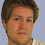## Friday, September 11, 2009

### Sugar Cube Friday

Just for fun, let's place an ant onto the corner of a sugar cube. The ant can only travel along the edges of the cube. The cube has sides all measuring 1 cm.

What is the total distance the ant can travel without retracing any part of its path?

1.The edges of a cube 1cm x 1cm x 1cm total 12cm, but I'm not sure if the ant would be able to trace all 12cm before crossing his path. In several attempts, I could only get 10 of the 12.

2.I am getting 9 cms.
www.guessthelogo.blogspot.com

3.A cube has eight vertices, each of which touches three edges. Except for his first and last move, the ant can only touch an even number of vertices at each edge. Therefore, the ants' optimal path will start at a vertex touching three used edges, pass through six vertices touching two used edges, and end at another vertex touching three used edges. This sums to eighteen, but we're counting each edge twice. Therefore, the upper bound is nine. A trivial realization of this bound is a path circumnavigating the top face, then the bottom face.

I'm pretty sure I'm right, at least. Can you describe your ten-edge path, Jered?

4.I'm getting 9 as well:

Three sides on top,
down one back wall,
three sides on bottom
up the back wall,
fourth side on top.

5.i got 9 too, two different ways, but still 9

6.The best I can do is 9, but I can't prove it. I'm pretty sure 9 is the answer, so if you have some way of explaining your answer of 10, please share it.

Thanks!

7.It's 9. See http://www.math.kent.edu/~soprunova/31011/hw11_sol.pdf . Construction of a cube requires at least 4 pieces of wire, so with a single piece, the best is 9 (12 edges minus 3 pieces missing).

8.TNT asked me to explain my proof a little better. I'm posting it here so it will help anyone else who's curious.

Okay, first, let's try to draw as long a path on the edges of a cube as possible, but add the constraint that we have to end up at the first place we started. (This is similar to a Eulerian circuit.) You can construct such a path using eight edges by connecting two parallel U shapes at the top (example).

Now notice that every vertex (corner) of the cube is connected to three edges, and in our circuit example, two of those edges are used for each vertex. This has to be the case, because every time you pass through a vertex, you use one edge to enter it and another to leave it. If we had four edges per vertex, we could pass through each twice, but on a cube, the maximum number of edges you can use for a vertex in the middle of your path is two. Since we've constrained ourselves to starting and ending at the same point, it doesn't matter where we start drawing the path, so we "pass through" every vertex, including our starting point.

For two used edges per corner and eight corners on a cube, we see sixteen corner-edge combinations. However, just like every corner in a cube is connected to three edges, every edge is connected to two corners. Thus, we've counted each edge twice, so we'll divide our result by two, giving us eight used edges. We've established that there can be no longer path when you start and end at the same point, and we've found an example of such a path, so we've proved that the longest possible circuit of the edges of a cube is eight edges.

How about when we eliminate the circuit constraint? Notice that any of the unused edges (those in green in my example image) can be used in a path if we start on one end of the new edge, cross the edge, then complete our original circuit, ending on the opposite end of the new edge. This is a valid nine-edge path, so we've proved that the maximum path length is at least nine edge.

Notice that during the circuit, we'll still pass through our starting position, so both our starting position and our ending position will connect to three used edges. Every other vertex will still connect to two used edges. Is there any way to use more edges? No, because we've used every edge connected to our starting and ending position, and every other vertex can only connect to an even number of edges (one for each entrance and one for each exit). Our path length formula is now (2 * 3 + 6 * 2) / 2, yielding nine. Thus, no path using each edge of a cube at most once can be more than nine edges long.

If no path can be longer than nine edge and we've found a nine-edge path, we've proved that the longest possible path on the edges of a cube is nine edges long.

I'd appreciate feedback, particularly from anyone who's found a ten-edge path.

9.Why not turn the cube into a net and work it out from there ?

10.Im getting 16 .....?

Leave your answer or, if you want to post a question of your own, send me an e-mail. Look in the about section to find my e-mail address. If it's new, I'll post it soon.

Please don't leave spam or 'Awesome blog, come visit mine' messages. I'll delete them soon after.

Enter your Email and join hundreds of others who get their Question of the Day sent right to their mailbox

The Lamplight Manor Puzz 3-D